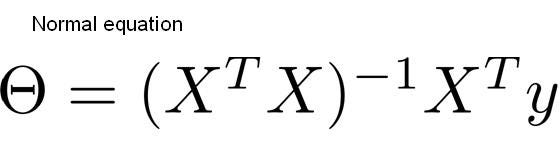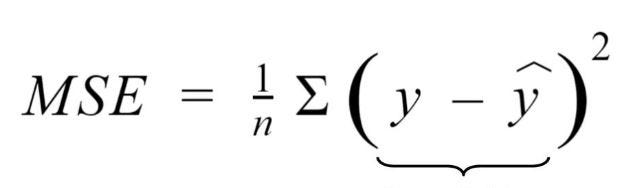# The Math behind Gradient Descent and the Normal Equation for Linear RegressionWhen i started my machine learning journey, the math was something that always intrigued me and still does. In this article, I will be going over the math behind Gradient Descent and the derivation behind the Normal linear Equation and then implementing them both on a dataset to get my coefficients. I for one believe that…

When I started my machine learning journey, math was something that always intrigued me and still does.

I for one believe that libraries such as scikit learn have indeed done wonders for us when it comes to implementing the algorithms but without an understanding of the maths that goes into making the algorithm, we are bound to make mistakes on complicated problems.

In this article, I will be going over the math behind Gradient Descent and the derivation behind the Normal linear Equation and then implementing them both on a dataset to get my coefficients.

A Linear Regression model. Red dots are the true y values and the blue line is the model line

## The Normal EquationLooks pretty straight forward, no? When I was getting started with Linear Regression and trying to get an understanding of the different ways to calculate the coefficients, The Normal Equation was by far my most favorite method to find coefficients but where does this equation come from? Well, let us take a look.

The first thing we have to understand is that the Mean Squared Error or MSE for short is a metric that measures how well our model performs. The lower the MSE, the closer our predictions are to the actual values of y.As can be seen in the equation, the lesser the difference between the actual and predicted values of y, the lower the MSE. And that is the goal, to have as low of an MSE as possible.

But can we use the MSE to find our coefficients? Yes, we can.

## Most popular Data Science and Machine Learning courses — July 2020

Most popular Data Science and Machine Learning courses — August 2020. This list was last updated in August 2020 — and will be updated regularly so as to keep it relevant

## Artificial Intelligence vs Machine Learning vs Data Science

Artificial Intelligence, Machine Learning, and Data Science are amongst a few terms that have become extremely popular amongst professionals in almost all the fields.

## AI(Artificial Intelligence): The Business Benefits of Machine Learning

Enroll now at CETPA, the best Institute in India for Artificial Intelligence Online Training Course and Certification for students & working professionals & avail 50% instant discount.

## Difference between Machine Learning, Data Science, AI, Deep Learning, and Statistics

In this article, I clarify the various roles of the data scientist, and how data science compares and overlaps with related fields such as machine learning, deep learning, AI, statistics, IoT, operations research, and applied mathematics.

## Gradient Descent for Data Science and Machine Learning

Gradient Descent for Data Science and Machine Learning. Solve Optimization Problems using Gradient Descent. You might not find it super exciting in and of itself, but it will enable us to do exciting things throughout the article, so bear with me.